Courses

# NCERT Solutions - Measures of Central Tendency Commerce Notes | EduRev

## Commerce : NCERT Solutions - Measures of Central Tendency Commerce Notes | EduRev

The document NCERT Solutions - Measures of Central Tendency Commerce Notes | EduRev is a part of the Commerce Course Economics Class 11.
All you need of Commerce at this link: Commerce

Page No 71:

Question 1: Which average would be suitable in the following cases?
(ii) Average intelligence of students in a class.
(iii) Average production in a factory per shift.
(iv) Average wage in an industrial concern.
(v) When the sum of absolute deviations from average is least.
(vi) When quantities of the variable are in ratios.
(vii) In case of open-ended frequency distribution.
(i) The demand for the average size of any readymade garment is the maximum. As, the modal value represents the value with the highest frequency, so the number of the average size to be produced is given by the Modal value.
(ii) Median will be the best measure for calculating the average intelligence of students in a class. It is the value that divides the series into two equal parts. So, number of students below and above the average intelligence can easily be estimated by median.
(iii) It is advisable to use mean for calculating the average production in a factory per shift. The average production is best calculated by arithmetic mean.
(iv) Mean will be the most suitable measure. It is calculated by dividing the sum of wages of all the labour by the total number of labours in the industry.
(v) When the sum of absolute deviations from average is the least, then mean could be used to calculate the average. This is an important mathematical property of arithmetic mean. The algebraic sum of the deviations of a set of n values from A.M. is 0.
(vi) Median will be the most suitable measure in case the variables are in ratios. It is least affected by the extreme values.
(vii) In case of open ended frequency distribution, Median is the most suitable measure as it can be easily computed. Moreover, the median value can be estimated even in case of incomplete statistical series.

Question 2(i): The most suitable average for qualitative measurement is
(a) arithmetic mean
(b) median
(c) mode
(d) geometric mean
(e) none of the above

Median is the most suitable average for qualitative measurement. This is because Median divides a series in two equal parts.

Page No 72:

Question 2(ii): Which average is affected most by the presence of extreme items?
(a) median
(b) mode
(c) arithmetic mean
(d) geometric mean
(e) harmonic mean
Arithmetic mean is the most affected by the presence of extreme items. It is one of the prime demerits of the arithmetic mean. It is easily distorted by the extreme values, and also the value of arithmetic mean may not figure out at all in the series.

Page No 72:

Question 2(iii): The algebraic sum of deviation of a set of n values from A.M. is
(a) n
(b) 0
(c) 1
(d) none of the above

Answer: The algebraic sum of deviation of a set of n values from A.M. is zero. This is one of the mathematical properties of arithmetic mean.

Question 3: Comment whether the following statements are true or false.
(i) The sum of deviation of items from median is zero.
(ii) An average alone is not enough to compare series.
(iii) Arithmetic mean is a positional value.
(iv) Upper quartile is the lowest value of top 25% of items.
(v) Median is unduly affected by extreme observations.

Answer: (i) The sum of deviation of items from median is zero. False
The statement is false. This mathematical property applies to the arithmetic mean that states that the sum of the deviation of all items from the mean is zero.
(ii) An average alone is not enough to compare series. True
An average indicates only the behaviour of a particular series. Therefore, in order to measure the extent of divergence of different items from the central tendency is measured by dispersion. So, average is not enough to compare the series.
(iii) Arithmetic mean is a positional value. False
This statement is false as mean is not a positional average, rather the statement holds true for median and mode. The calculation of median and modal values is based on the position of the items in the series, i.e. why these are also termed as positional averages.
(iv) Upper quartile is the lowest value of top 25% of items. True
The value that divides a statistical series into four equal parts, the end value of each part is called quartile. The third quartile or the upper quartile has 75 % of the items below it and 25 % of items above it,
(v) Median is unduly affected by extreme observations. False
This statement is true for Arithmetic mean. Arithmetic mean is most affected by the presence of extreme items. It is one of the prime demerits of the arithmetic mean. It is easily distorted by the extreme values, and also the value of arithmetic mean may not figure out at all in the series.

Question 4: If the arithmetic mean of the data given below is 28, find (a) the missing frequency, and (b) the median of the series: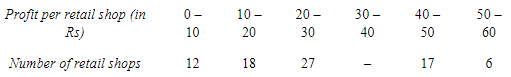(i) Let the missing frequency be f1
Arithmetic Mean = 28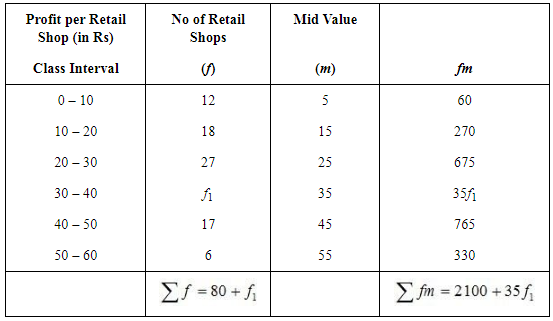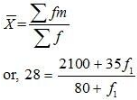or, 2240 + 28f1 = 2100 + 35f1
or, 2240 – 2100 = 35f1 – 28f1
or, 140 = 7f1
f1 = 20
(ii)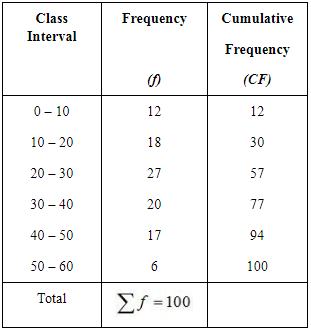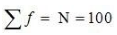So, the Median class = Size of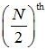item
= 50th item
50th item lies in the 57th cumulative frequency and the corresponding class interval is 20 – 30.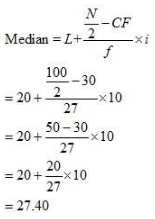Question 5: The following table gives the daily income of ten workers in a factory. Find the arithmetic mean.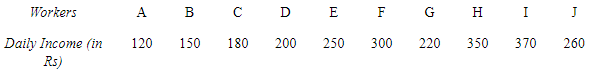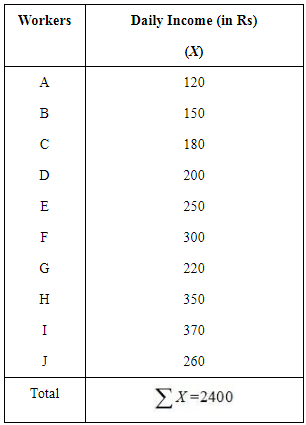N = 10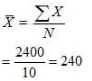Arithmetic mean = Rs 240

Question 6: Following information pertains to the daily income of 150 families. Calculate the arithmetic mean.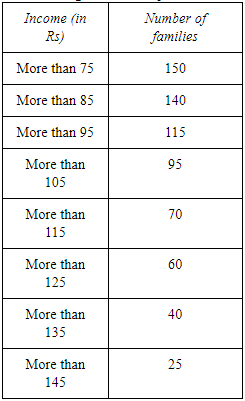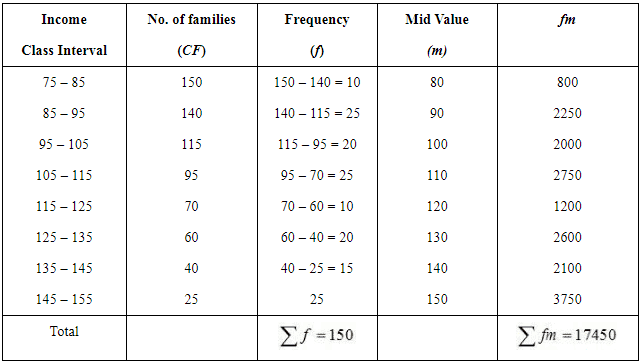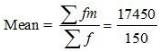= Rs 116.33

Page No 73:

Question 7: The size of land holdings of 380 families in a village is given below. Find the median size of land holdings.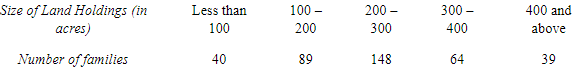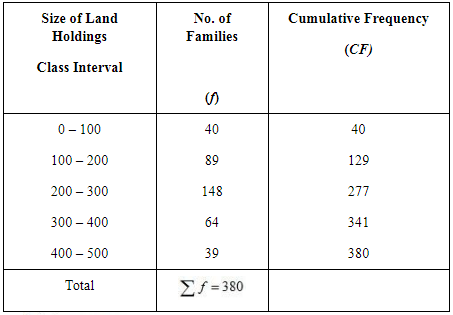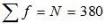So, the Median class = Size of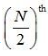item = 190th item
190th item lies in the 129th cumulative frequency and the corresponding class interval is 200 – 300.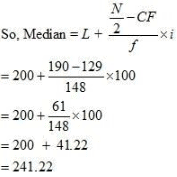Median size of land holdings = 241.22 acres

Question 8: The following series relates to the daily income of workers employed in a firm. Compute (a) highest income of lowest 50% workers (b) minimum income earned by the top 25% workers and (c) maximum income earned by lowest 25% workers.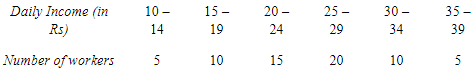(Hint: Compute median, lower quartile and upper quartile)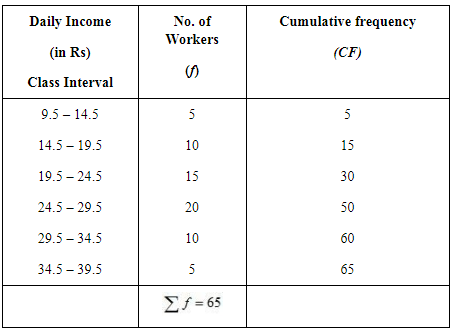(a) Highest income of lowest 50% workers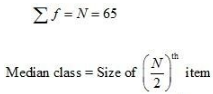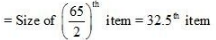32.5th item lies in the 50th cumulative frequency and the corresponding class interval is 24.5 – 29.5.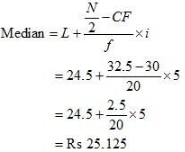(b) Minimum income earned by top 25% workers

In order to calculate the minimum income earned by top 25% workers, we need to ascertain Q3.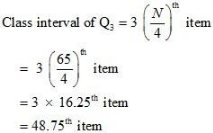48.75th item lies in 50th item and the corresponding class interval is 24.5 – 29.5.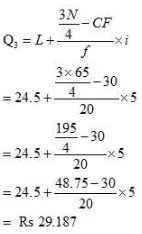(c) Maximum  income earned by lowest 25% workers

In order to calculate the maximum income earned by lowest 25% workers, we need to ascertain Q1.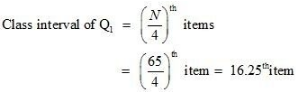16.25th item lies in the 30th cumulative frequency and the corresponding class interval is 19.5 – 24.5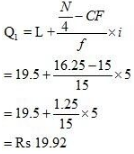Question 9: The following table gives production yield in kg. per hectare of wheat of 150 farms in a village. Calculate the mean, median and mode values.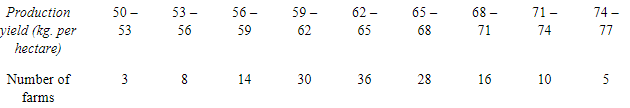(i) Mean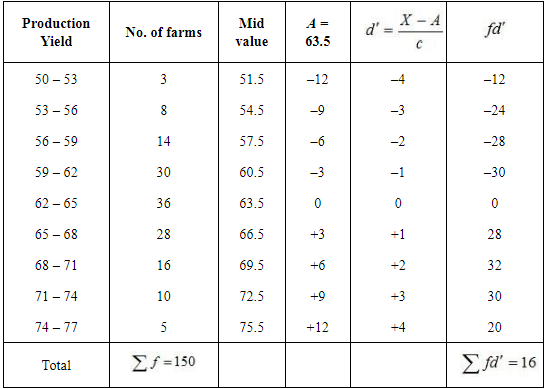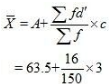= 63.5 + 0.32
= 63.82 kg per hectare
(ii) Median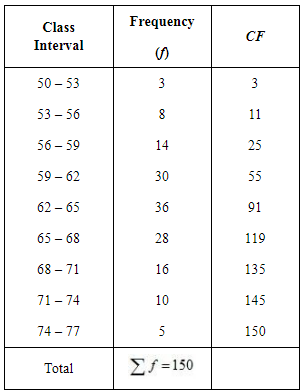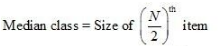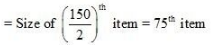75th item lies in the 91st cumulative frequency and the corresponding class interval is 62 – 65.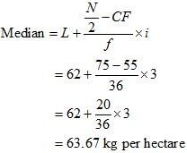(iii) Mode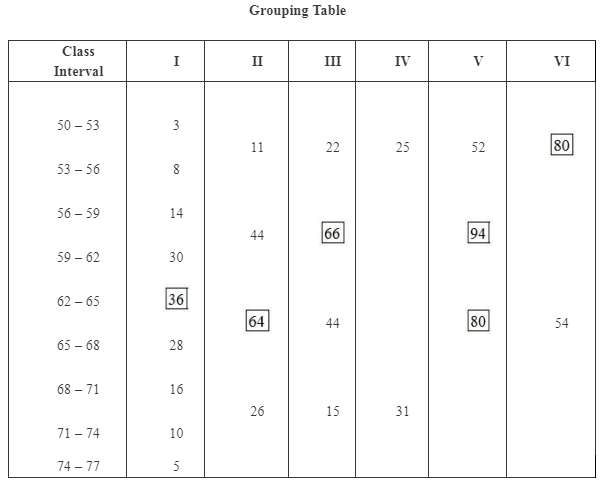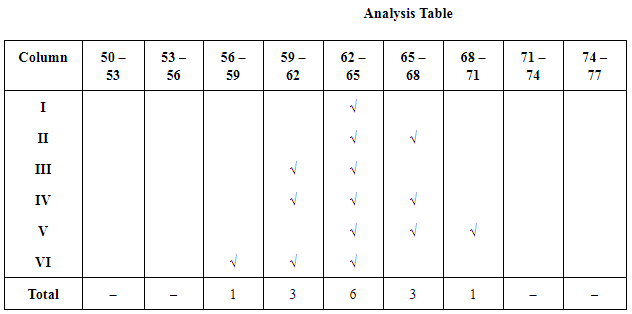Modal class = 62 – 65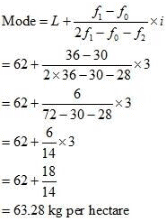Offer running on EduRev: Apply code STAYHOME200 to get INR 200 off on our premium plan EduRev Infinity!

,

,

,

,

,

,

,

,

,

,

,

,

,

,

,

,

,

,

,

,

,

;